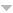# Publications

Found 54 results
Author Title Type [ Year]
Filters: First Letter Of Last Name is R and Author is Rosales, J. C.  [Clear All Filters]
2017
Parametrizing Arf numerical semigroups. J. Algebra Appl.. 2017;16:1750209, 31.Edit
2009
Numerical semigroups. Vol 20 Springer, New York 2009.Edit
2006
The catenary and tame degree in finitely generated commutative cancellative monoids. Manuscripta Math.. 2006;120:253-264.Edit
On the Frobenius number of a proportionally modular Diophantine inequality. Port. Math. (N.S.). 2006;63:415-425.Edit
Numerical semigroups with maximal embedding dimension [\refcno 2056070]. In: Focus on commutative rings research. Nova Sci. Publ., New York; 2006. 4. p. 47-53p. Edit
Presentations of finitely generated cancellative commutative monoids and nonnegative solutions of systems of linear equations. Discrete Appl. Math.. 2006;154:1947-1959.Edit
Presentations of finitely generated cancellative monoids and natural solutions of linear systems of equations. In: Fifth Conference on Discrete Mathematics and Computer Science (Spanish). Vol 23. Univ. Valladolid, Secr. Publ. Intercamb. Ed., Valladolid; 2006. 2. p. 217-224p. (Ciencias (Valladolid); vol 23).Edit
2005
$k$-factorized elements in telescopic numerical semigroups. In: Arithmetical properties of commutative rings and monoids. Vol 241. Chapman & Hall/CRC, Boca Raton, FL; 2005. 2. p. 260-271p. (Lect. Notes Pure Appl. Math.; vol 241).Edit
Modular Diophantine inequalities and numerical semigroups. Pacific J. Math.. 2005;218:379-398.Edit
Numerical semigroups with a monotonic Apéry set. Czechoslovak Math. J.. 2005;55(130):755-772.Edit
Pseudo-symmetric numerical semigroups with three generators. J. Algebra. 2005;291:46-54.Edit
2004
Arf numerical semigroups. J. Algebra. 2004;276:3-12.Edit
Atomic commutative monoids and their elasticity. Semigroup Forum. 2004;68:64-86.Edit
Fundamental gaps in numerical semigroups. J. Pure Appl. Algebra. 2004;189:301-313.Edit
Fundamental gaps in numerical semigroups with respect to their multiplicity. Acta Math. Sin. (Engl. Ser.). 2004;20:629-646.Edit